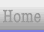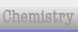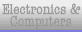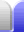# Factorising Confusion

Factorising can be a very useful and powerful technique, but it's possible to make mistakes which can lead to confusion.

### 2 + 2 = 5

Start with a number, say –20, and make an equation by letting it equal itself, then rearrange each side.

–20 = –20

16 – 36 = 25 – 45

42 – 4 x 9 = 52 – 5 x 9

(2 + 2)2 – (2 + 2) x 9 = 52 – 5 x 9

(2 + 2)2 – (2 + 2) x 9 + 81/4 = 52 – 5 x 9 + 81/4

Each side is now a perfect square, so can be factorised. We'll take several steps to rearrange it so it's easier to recognise as a perfect square.

(2 + 2)2 – (2 + 2) x 9 + / = 52 – 5 x 9 + /

(2 + 2)2 – (2 + 2) x 9 + (9/2)2 = 52 – 5 x 9 + (9/2)2

(2 + 2)2 – 2 x (2 + 2) x 9/2 + (9/2)2 = 52 – 2 x 5 x 9/2 + (9/2)2

This is easier to see how it can factorise, because each side is in the form a2 + 2ab + b2.

((2 + 2) – 9/2)2 = (5 – 9/2)2

From there, we just need to take the square root of each side and cancel the common term.

(2 + 2) – 9/2 = 5 – 9/2

2 + 2 = 5

What went wrong? This error is not something that just happens in factorising. It's a good example of what can happen when we're not paying attention.